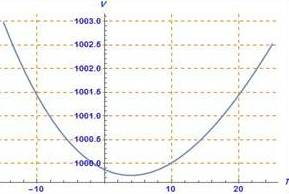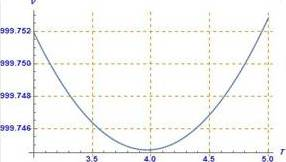# the temperature at which the volume of 1 k g of water is a minimum### Precalculus: Mathematics for Calcu...

6th Edition
Stewart + 5 others
Publisher: Cengage Learning
ISBN: 9780840068071### Precalculus: Mathematics for Calcu...

6th Edition
Stewart + 5 others
Publisher: Cengage Learning
ISBN: 9780840068071

#### Solutions

Chapter 2.3, Problem 54E
To determine

## To find : the temperature at which the volume of 1kg of water is a minimum

Expert Solution

### Explanation of Solution

Given information : The question is

Between 0C and 30C , the volume V of 1kg water at a temperature T is given V=999.870.06426T+0.0085043T20.0000679T3

Graph : The graph of V as a function of T is shown

There appears to be minimum between T=1C and T=5C

Using the maximum command,

The maximum value of V is about 999.745 , and it occurs when T=3.966CInterpretation : The temperature at which the volume of 1kg of water is a minimum is T=3.966C because the maximum value of V is about 999.745

### Have a homework question?

Subscribe to bartleby learn! Ask subject matter experts 30 homework questions each month. Plus, you’ll have access to millions of step-by-step textbook answers!